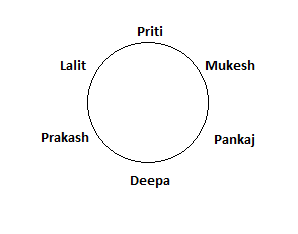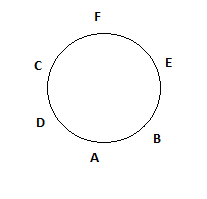0
2105

# SSC CHSL Seating Arrangement Questions

Download Top-20 Seating Arrangement questions for SSC CHSL exam 2020. Most important  English questions based on asked questions in previous exam papers for SSC CHSL.

Instructions

Study the following information carefully and answer the question given below :

Nine persons – A, B, C, D, E, F, G, H and I – are sitting around a circular table facing the centre and at equal distance but not necessarily in the same order. B is immediate neighbour of neither I nor C. A is sitting third to the left of B. F is sitting third to the right of C. G is immediate neighbour of neither F nor A. D is sitting third to to left of I. H is an immediate neighbor of both A and G.

Question 1: Four of the following five pairs are alike in a certain way based on above seating arrangement and hence form a group. Which one of the following ‘’does not’’ belong to that group?

a) AC

b) DB

c) EI

d) GI

e) HA

Question 2: Starting from A, if all the persons are made to sit in the alphabetical order in clock – wise direction, the position of how many (excluding A) will remain unchanged?

a) None

b) One

c) Two

d) Three

e) More than three

Question 3: What is the position of G with respect to F?

a) Third to right

b) Fourth to right

c) Third to the left

d) Fourth to the left

e) Fifth to the right

Question 4: Who among the following is sitting third to the left of H?

a) C

b) A

c) I

d) G

e) E

Question 5: Who among the following sits exactly between G and I?

a) B

b) E

c) D

d) C

e) F

Question 6: Which of the following statements is ‘’NOT TRUE’’ with respect to the given seating arrangement?

a) C is an immediate neighbor of both A and D

b) H is sitting second to the left of C

c) B is sitting exactly between D and F

d) A is sitting second to the right of G

e) All are true

Instructions

Study the following information carefully and answer the questions given below :

Ten persons – L, M, N, O, P, Q, R, S, T and U – are standing in a straight line facing north, but not necessarily in the same order. Four persons are standing between Q and S. P is standing at one of the extreme ends. L is standing to the immediate right of Q. L is standing second to the left of P. U is standing second to the right of S and second to the left of N. Three persons are standing between R and M. O is not standing at any extreme end of the line. M is far away from O than that of P.

Question 7: Who among them standing at extreme left end ?

a) R

b) T

c) S

d) Q

e) P

Question 8: If all persons are made to stand in the alphabetical order from right to left starting from L, the positions of how many persons (excluding L) will remain unchanged?

a) One

b) Two

c) Three

d) Four

e) None

Question 9: How many persons standing between M and O ?

a) None

b) One

c) Two

d) Three

e) Five

Question 10: Which of the following pairs represents the immediate neighbours of S ?

a) OU

b) RU

c) TU

d) TO

e) NO

Question 11: Four of the following five are alike in a certain way based on their positions in the above arrangement and hence form a group. Which is the one that ‘’does not’’ belong to that group ?

a) ST

b) ML

c) UR

d) NR

e) LQ

Question 12: Who among the following is standing fourth to the left of M ?

a) R

b) N

c) L

d) U

e) S

Question 13: Six friends are sitting in a circle and are facing the centre of the circle. Deepa is between Prakash and Pankaj. Priti is between Mukesh and Lalit. Prakash and Mukesh are opposite to each other. Who is sitting opposite to Priti ?

a) Mukesh

b) Deepa

c) Pankaj

d) Lalit

Question 14: Six friends are sitting in a circle and are facing the centre of the circle. Deepa is between Prakash and Pankaj. Priti is between Mukesh and Lalit. Prakash and Mukesh are opposite to each other. Who is sitting opposite to priti ?

a) Prakash

b) Deepa

c) Pankaj

d) Lalit

Question 15: (i) Six friends A, B, C, D, E and F are seated in a circle facing each other.
(ii) A is between D and B and F is between C and E
(iii) C is the third to the left of B
Which of the following is the position of A in relation to F ?

a) Second to the left

b) Second to the right

c) third to the right

d) Fourth to the left

Question 16: 22 boys are standing in front of Kunal in a queue. 12 boys are standing to the back of Rohit in the same queue. If total number of boys is 30 then the number of boys standing in between Kunal and Rohit is

a) 3

b) 4

c) 6

d) 5

Question 17: 32 boys are standing in front of X in a queue. Y is standing in the 19th position from the back in the same queue.If total number of boys is 45 then the number of boys standing in between X and Y is

a) 3

b) 5

c) 6

d) 4

Question 18: In a class of 72 children, children are seated in rows and columns in such a way that the number of children in each row is 12.5% more than the number of children in each column. How many children are there in each row ?

a) 9

b) 8

c) 18

d) 12

Instructions

There are 15 students in a class who are equally distributed 5 benches forming a rectangular array of students.
Boys and Girls sit in separate benches Shravya is seated in one corner of first bench Jayant is diagonally opposite to her in the last bench.Aruna is in between Shravya and Kavya in one bench. Kavya asked the girl immediately behind her Shilpa for a pencil and Shilpa borrowed a pencil from the boy immediately behind her Anil, and gave it to Kavya Sunil is in between Anil and Jayant. After some time Shilpa goes out and Shravya sits in place of her but as Geeta did not want to sit beside Shravya Geeta swaps her place with Sweta.

Question 19: How many girls are there in the Class after Shilpa left the Class ?

a) 8

b) 5

c) 6

d) 11

Question 20: Before Shilpa left the Class how was the seating placement for Jayant and Shilpa ?

a) Jayant was exactly behind Shilpa

b) Jayant and Shilpa were diagonally opposite

c) Two students were there between Jayant and Shilpa diagonally

d) Jayant is two students behind Shilpa.

The data given to us is:
Deepa is between Prakash and Pankaj. Priti is between Mukesh and Lalit. Prakash and Mukesh are opposite to each other.

This can be intrepreted in diagrammatic form as:Therefore, Deepa is sitting opposite to Priti.

The data given to us is:
Deepa is between Prakash and Pankaj. Priti is between Mukesh and Lalit. Prakash and Mukesh are opposite to each other.

This can be intrepreted in diagrammatic form as:Therefore, Deepa is sitting opposite to Priti.

The data given to us is:
(i) Six friends A, B, C, D, E and F are seated in a circle facing each other.
(ii) A is between D and B and F is between C and E
(iii) C is the third to the left of B

Now, we can represent this in diagrammatic form as:Hence, A is fourth to the left of A.
Therefore, option D is the correct answer.

22 boys are standing in front of Kunal in a Queue 12 boys are standing to the back of Rohit
Total boys = 30.
So, rank of Kunal in the queue is 22+1 = 23
Rank of Rohit in the queue is 30 – 12 = 18
Hence, the number of boys between Rohit and Kunal in the queue is 23 – 18 – 1 = 4

There are 32 boys ahead of X and 26 ahead of Y. Since Y is also ahead of X, there are 31 other boys ahead of X. ie there are 5 boys between X and Y.

let the number of children in each column be x.Thus, the number of children in each row is 1.125

given, total number of children=72=x(1.125x)

∴x=8.

thus, number of children in a row=1.125x=9